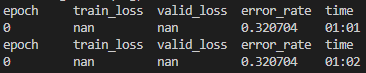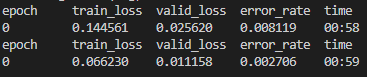# [WINDOWS, MULTI-PROCESS, CUDA] fastai-v2, NaN values during resnet finetune / train / or using normalize with GPU

Please refer to the program below:

``````from fastai.vision.all import *
from ilearn.utl.misc import untar_data_ex
from imind.globals.iconfigparsers import EnvConfig

def label_func(f):
return f.isupper()

def get_data(url, presize, resize):
path = untar_data_ex(url, base=Path(EnvConfig.section('DATA_PATHS').FASTAI_DATA))
files = get_image_files(path/"images")
dls = ImageDataLoaders.from_name_func(path, files, label_func, item_tfms=Resize(224), num_workers=1) # verbose=True
return dls

if __name__ == "__main__":
multiprocessing.set_start_method('spawn')  # default for windows
dls = get_data(URLs.PETS, 224, 224)

# Pre-trained model
learn = vision_learner(dls, resnet34, metrics=error_rate) # normalize=True by default
learn.fine_tune(1, 3e-3)
``````

Program Output:Problem:
Platform: Windows 10x64, GPU: Nvidia 1080Ti

• resnet34 (pre-trained) model uses pre-trained weights hence `vision_learner` calls Normalize.from_stats(…) with pre-trained resnet relevant mean, and std which will be moved to GPU (if cuda is available)

• While feeding the batches from multiple processes (num_workers=1 or > 1), the batches are found to contain nan due to the division mean and std being 0 (ref Normalize.encodes)

• This is because initially, mean and std are moved to GPU when Normalize.from_stats(…) is called, and by the time batches are formed from multiple processes and follow Normalize.encodes(…), the mean and std are taken as 0 in GPU.

• This problem should have something to do with CUDA and accessing GPU tensors (mean, std) with multiple windows processes, according to my initial study.

• Once I use my own Normalize2 class as shown below (keeping mean, std in the memory and moving them to GPU every time encodes is called - highly inefficient), it works.If anyone is familiar with or having the same issue, please let me know. Your suggestions / ideas are highly appreciated. Thank you.

Ref: fastai-v2 Normalize Class

``````class Normalize(DisplayedTransform):
"Normalize/denorm batch of `TensorImage`"
parameters,order = L('mean', 'std'),99
def __init__(self, mean=None, std=None, axes=(0,2,3)): store_attr()

@classmethod
def from_stats(cls, mean, std, dim=1, ndim=4, cuda=True): return cls(*broadcast_vec(dim, ndim, mean, std, cuda=cuda))

if self.mean is None or self.std is None:
x,*_ = dl.one_batch()
self.mean,self.std = x.mean(self.axes, keepdim=True),x.std(self.axes, keepdim=True)+1e-7

def encodes(self, x:TensorImage): return (x-self.mean) / self.std  # <= mean, std here is 0
def decodes(self, x:TensorImage):
f = to_cpu if x.device.type=='cpu' else noop
return (x*f(self.std) + f(self.mean))

_docs=dict(encodes="Normalize batch", decodes="Denormalize batch")
``````

Temporary Fix (highly inefficient):

``````#!/usr/bin/env python
# coding: utf-8

from fastai.vision.all import *
from ilearn.utl.misc import untar_data_ex
from imind.globals.iconfigparsers import EnvConfig

def label_func(f):
return f.isupper()

class Normalize2(DisplayedTransform):
"Normalize/denorm batch of `TensorImage`"
# parameters,order = L('mean', 'std'),99
# TfmdDL(DataLoader): to() method will put the `parameters` above, to GPU  # FIX: (stop moving `parameters` to GPU)

order = 99

def __init__(self, mean=None, std=None, axes=(0,2,3)):
store_attr()
pass

@classmethod
def from_stats(cls, mean, std, dim=1, ndim=4, cuda=True):
return cls(*broadcast_vec(dim, ndim, mean, std, cuda=cuda))

if self.mean is None or self.std is None:
x,*_ = dl.one_batch()
self.mean,self.std = x.mean(self.axes, keepdim=True),x.std(self.axes, keepdim=True)+1e-7

def encodes(self, x:TensorImage):
[mean, std] = broadcast_vec(1, 4, self.mean, self.std)  # FIX: Move mean, std to GPU before normalizing x
return (x-mean) / std

def decodes(self, x:TensorImage):
[mean, std] = broadcast_vec(1, 4, self.mean, self.std)  # FIX: Move mean, std to GPU

f = to_cpu if x.device.type=='cpu' else noop
return (x*f(std) + f(mean))

def get_data(url, presize, resize):
path = untar_data_ex(url, base=Path(EnvConfig.section('DATA_PATHS').FASTAI_DATA))
files = get_image_files(path/"images")
dls = ImageDataLoaders.from_name_func(path, files, label_func, item_tfms=Resize(224),
batch_tfms=Normalize2.from_stats([0.485, 0.456, 0.406], [0.229, 0.224, 0.225], cuda=False),  # FIX: cuda=False, store in memory (do not move to GPU)
num_workers=1)
return dls

if __name__ == "__main__":
multiprocessing.set_start_method('spawn')  # default for windows
dls = get_data(URLs.PETS, 224, 224)

# Pre-trained model
learn = vision_learner(dls, resnet34, normalize=False, metrics=error_rate)  # FIX: normalize=False
learn.fine_tune(1, 3e-3)
``````My fastai-v2 platform info:

``````=== Software ===
python        : 3.9.12
fastai        : 2.7.9
fastcore      : 1.5.6
fastprogress  : 1.0.2
torch         : 1.12.0
nvidia driver : 516.59
torch cuda    : 11.3 / is available
torch cudnn   : 8302 / is enabled

=== Hardware ===
nvidia gpus   : 2
torch devices : 2
- gpu0      : NVIDIA TITAN X (Pascal)
- gpu1      : NVIDIA GeForce GTX 1080 Ti

=== Environment ===
platform      : Windows-10-10.0.19044-SP0
conda env     : base
python        : D:\anaconda3\python.exe
sys.path      : g:\OneDrive\chath_curtin\OneDrive - Curtin\Research\dev\python\DLN\tutorials\02_dl\01_pytorch\frameworks\01_fastai\fastai\Bugs\1
G:\OneDrive\chath_curtin\OneDrive - Curtin\Research\dev\python\DLN
G:\OneDrive\chath_curtin\OneDrive - Curtin\Research\dev\python\_installers\fastlogging-master
D:\anaconda3\python39.zip
D:\anaconda3\DLLs
D:\anaconda3\lib
D:\anaconda3
C:\Users\chath\AppData\Roaming\Python\Python39\site-packages
C:\Users\chath\AppData\Roaming\Python\Python39\site-packages\fastlogging-1.0.0-py3.9-win-amd64.egg
D:\anaconda3\lib\site-packages
D:\anaconda3\lib\site-packages\win32
D:\anaconda3\lib\site-packages\win32\lib
D:\anaconda3\lib\site-packages\Pythonwin
``````

I’ve really no clue, but do notice here
that the `if __name__ == "__main__" ` line
is followed by a single line, calling a function that would wrap your lines following `if __name__ == "__main__" `

p.s. I like your comprehensive presentation of the issue. Hope someone can help further.

p.p.s. Try using WSL instead. Some of the Live Coding Sessions describe how to set up a Jupyter server on WSL. This is how I use FastAI locally (on a laptop so CPU only).

``````import torch